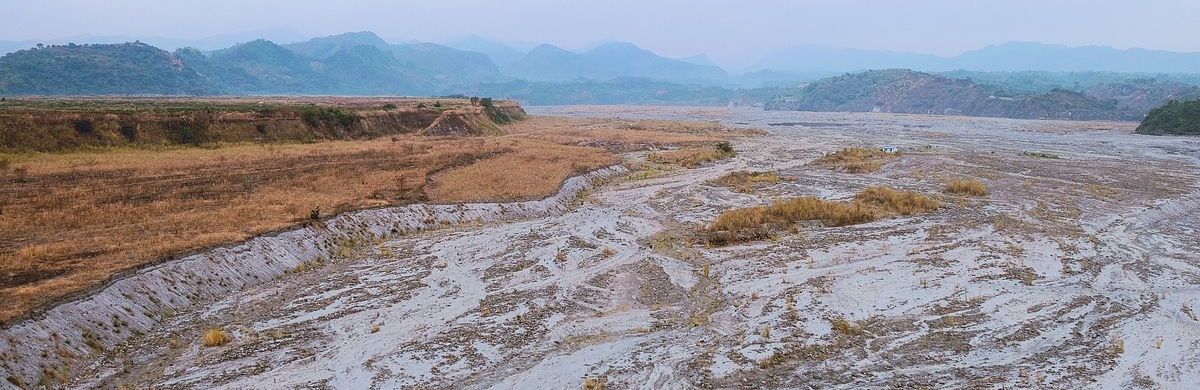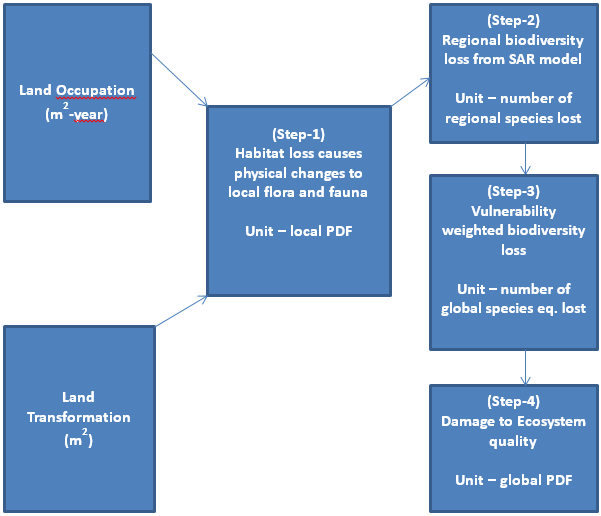a spatially differentiated life cycle impact assessment method# Land stress

#### Ecosystem quality

###### Land stress

Land use is a main driver of global biodiversity loss (MES 2005). Within a product’s life cycle, the land use impacts can represent a significant portion of their total environmental burden. The impact assessment approach proposed by Chaudhary et al. (2015) using countryside species-area relationship (SAR) is used for calculating ecoregion specific marginal and average characterization factors (CFs) for biodiversity loss for both land occupation and transformation.

#### Cause-effect pathway#### Modeling approach

Two different sets of CFs are available: (1) marginal CFs, which are typically used in LCA to address impacts of additional land use and (2) average CFs, which are used to assess total impacts of land use within a region. We provide CFs using the countryside SAR (Pereira & Daily 2006) for five taxa (mammals, birds, reptiles, amphibians, and vascular plants) and six land use types (annual crops, permanent crops, pastures, urban, extensive forestry and intensive forestry). Definitions of each of the land use types are taken from Koellner et al. (2013). The CFs are weighted with vulnerability scores of each taxa which is a function of the geographic range of each species and a threat level. Ecoregions are used as spatial units because their boundaries approximate the original extent of natural ecosystems before major land use changes and distinct communities of species are known to exist within a given ecoregion (Olson et al 2001).

#### Value choices

The value choice in the modelling of the land transformation impacts is the time horizon. As explained in the section 1.5 of framework chapter, the further away in time the impact is, the more uncertain its value is, (i.e. lower the level of robustness). We calculated two sets of transformation CFs. The user can choose between short-term “core” CFs (i.e. those calculated using the 100 year time horizon cut-off) or the precautionary CFs (calculated using total recovery times).

#### Spatial variability

The method was applied to 804 ecoregions with varying sizes, resulting in a global coverage. A global average is not considered meaningful but provided for background processes. Country averages are provided based on the share of ecoregions within them.

#### Characterisation factors

The species lost Slost,j,g per taxonomic group g due to cumulative land use in an ecoregion i>j is given by countryside SAR:

$$\mathsf{S_{lost,j,g}^{countryside}=S_{org,g,j}-S_{new,g,j}=\\=S_{org,g,j}-S_{org,g,j}\left(\frac{A_{new,j}+\sum_{i=1}^nh_{g,i,j}\cdot A_{i,j}}{A_{org,j}}\right)^{Z_j}}$$

where Anew is the remaining habitat area, Sorg is the number of species occurring in the original habitat area Aorg, Ai,j is the area of different land use types within an ecoregion, zj is the SAR exponent and hg.i.j is the affinity of taxa to land use type i.
This regional damage is then allocated to the different land use types i in the ecoregion j according to their area share pi.j and the species affinity to them through an allocation factor ai,j. The regional characterization factors (CFs) for occupation of each land use type are calculated by multiplying the species lost per region j with the corresponding allocation factor ai,j and dividing this by the area occupied by the land use type Ai,j.

$$\mathsf{CF_{occ,g,i,j}=\frac{S_{lost,g,j}\cdot a_{i,j}}{A_{i,j}}}$$

For land transformation the CFs are calculated as a multiplication of CFocc,g,i,j with half the regeneration time.

$$\mathsf{CF_{trans,g,i,j}=0.5\cdot CF_{occ,g,i,j}\cdot t_{reg,g,i,j}}$$

The regional CFs calculated above are multiplied by vulnerability score (0<VS<1) of that taxa in that ecoregion to obtain weighted-CFs for both land occupation and transformation.

$$\mathsf{CF_{weighted,g,i,j}=CF_{unweighted,g,i,j}\cdot VS_{g,j}}$$

The unit of weighted occupation CF is species eq. lost/m2 while the unit of weighted transformation CFs is species eq. lost · years/m2.

###### References

Millennium Ecosystem Assessment. 2005. Millennnium Ecosystem Assessment. Ecosystems and human well-being: biodiversity synthesis. World Resources Institute, Washington.
Olson D, Dinerstein E, Wikramanayake E, Burgess N, Powell G, Underwood E, D’Amico J, Itoua I, Strand H, Morrison J, Loucks C, Allnutt T, Ricketts T, Kura Y, Lamoreux J, Wettengel W, Hedao P, Kassem K. 2001. Terrestrial ecoregions of the worlds: A new map of life on Earth. BioScience, 51, 933− 938.
Pereira H, Daily G. 2006. Modeling biodiversity dynamics in countryside landscapes. Ecology, 87, 1877−1885.